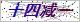网站首页  ◇  产品展示    ◇  仪表备品备件 ◇   电磁阀 > Z689=118=024溢流容器电磁阀
•••# 溢流容器电磁阀

Products

### 产品概述

689=118=024型  硅、磷表溢流容器电磁阀Z-689=118=024硅、磷表溢流容器电磁阀是德国burkert公司制造商生产的产品，配套与9210/9211硅磷表。9210硅酸根/磷酸根分析仪备件

9210硅表CPU板带显示        09200=A=1000
9211磷表CPU板带显示        09200=A=1011

RS485板（MODBUS）        09125=A=0485/2485
92XX内部总线通讯板        09200=A=5500
2米内部连接电缆/4米/7米        370=302=034/304/307
921X测量板（0~1000ppb-SiO2/0~5ppm-PO4）        0921X=A=1500
9210硅表测量板（0~5000ppb-SiO2）        09210=A=1510
9211磷表测量板（0~50ppm-PO4）        09210=A=1520

O-型圈（流速调节螺钉）,4×1mm        35600905040

O-型圈（液位检测器）,38×2mm        35609935308
样水电磁阀,（溢流容器）        689=118=024
921X连接板（混合器/加热器）        09210=A=1234
921X混合器        09210=A=0400
磁性搅拌棒         226=003=013
加热元件        09097=C=0560
Pt100温度探头        35900040780
921X光缆        09210=A=0500
921X光学镜        09210=C=0340
921X镜子隔圈        09210=C=0330
O-型圈（光学镜）,22×1mm        35609905220
方形盖（光度计池）        09210=C=0310
试剂管,1,6×3,2mm        590=050=060
试剂管内插管,0,8×1,6mm
试剂泵        695=114=001
校准/冲洗泵        695=004=004
921X2升试剂桶        495=020=001
921X试剂管沉头        09210=A=0600
9210硅表化学试剂（不含硫酸）        09210=C=7000
9211磷表化学试剂（0~5ppmPO4,不含硫酸）        09211=C=7000
9211磷表化学试剂（0~50ppmPO4,不含硫酸）        09211=C=7001
9210硅表药剂（一年用量）        09210=A=0507

9210硅表测量槽的主要作用是提供水样和试剂进行反应和测量的场所。测量槽主要分为上下两个部分，上面部分为不锈钢材质，主要包括光学镜和小磁棒，还有光纤光源。下面主要是温控部分。硅分析仪平时运行时，由于水质的原因(我们测量的是脱盐水站阳床出水)，会对测量槽造成污染。主要就是污染光学镜影响光路。另外由于小磁棒的磁性，从而吸附水中磁性污染物(磁性氧化铁)所以要求仪表维护人员要定期清洗测量槽，以保证仪表正常测量。

### 留言框

• #### 验证码：请输入计算结果（填写阿拉伯数字），如：三加四=7

13865908184